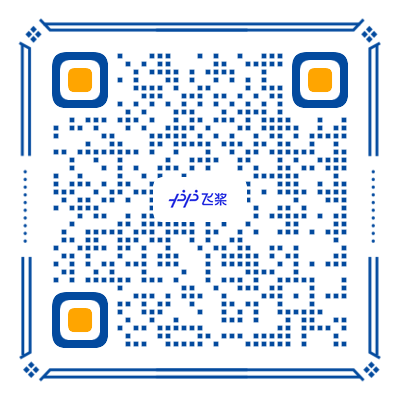\u200E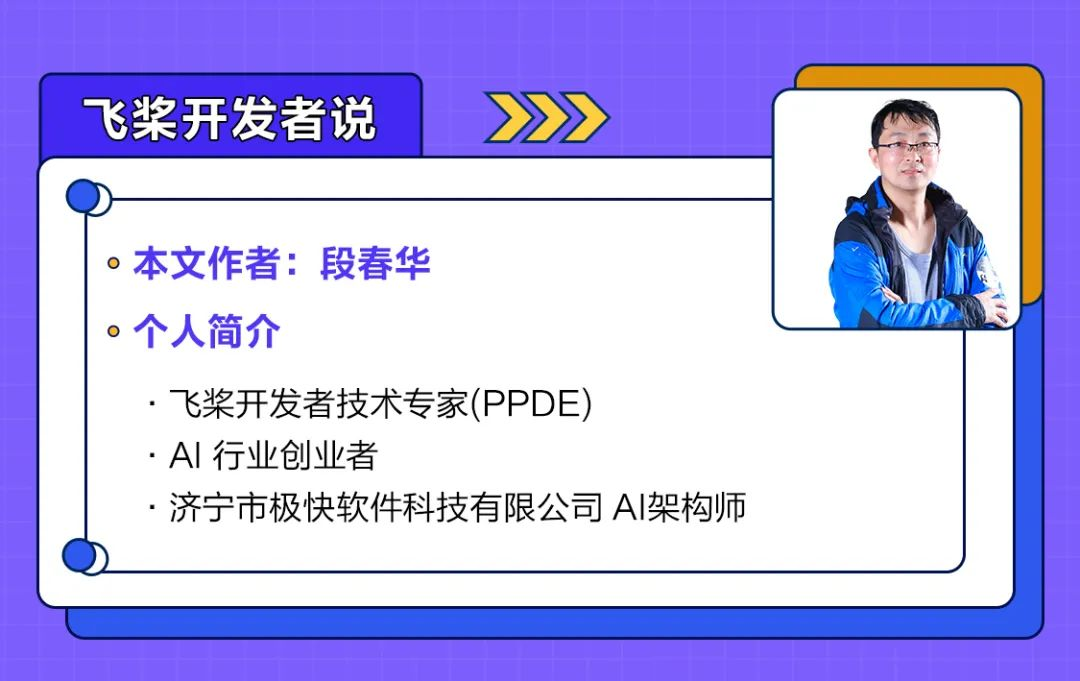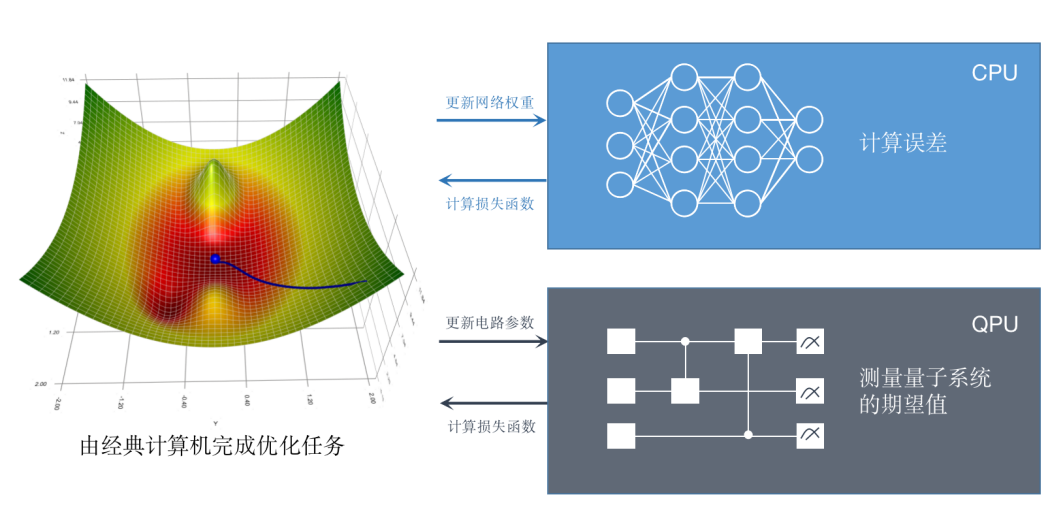图1 经典神经网络与量子神经网络对比图

QNN 通常可以表示为一些单量子比特旋转门和双比特门的组合。其中一个可以高效利用硬件的架构是只包含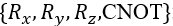这四种量子门的模板，它们很容易在近期的超导量子设备上实现。以下图为例：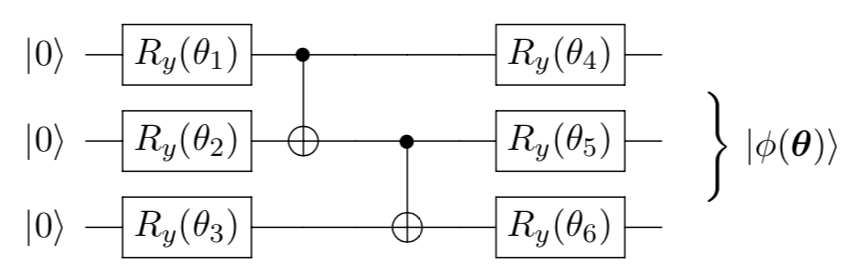图2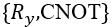组成的简单量子神经网络，共计6个参数

``# 引入必要的包import numpy as npimport paddlefrom paddle_quantum.circuit import UAnsatz# 随机初始化参数theta = np.random.rand(6)   # 我们需要将 Numpy array 转换成飞桨中的 Tensortheta = paddle.to_tensor(theta)# 初始化量子电路num_qubits = 3cir = UAnsatz(num_qubits)# 添加单比特旋转门cir.ry(theta, 0)cir.ry(theta, 1)cir.ry(theta, 2)# 添加两比特门cir.cnot([0, 1])cir.cnot([1, 2])# 添加单比特旋转门cir.ry(theta, 0)cir.ry(theta, 1)cir.ry(theta, 2)print('图中量子神经网络结构和参数为:')print(cir) ``

https://aistudio.baidu.com/aistudio/competition/detail/70

https://qml.baidu.com/quick-start/quantum-neural-network.html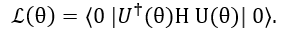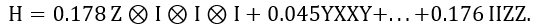``# 导入相关库import numpy as npimport paddlefrom paddle_quantum.circuit import UAnsatzfrom paddle_quantum.utils import pauli_str_to_matrixfrom paddle_quantum.VQE.chemistrysub import H2_generator# 读取内置好的泡利字符串形式的氢分子哈密顿量Hamiltonian, N = H2_generator()    # N 表示量子比特个数，这里为4# 超参数设置ITR = 80     # 设置训练的总迭代次数LR = 0.4     # 设置学习率D = 2        # 设置量子神经网络中重复计算模块的深度 Depth   # 把记录的关于哈密顿量的转化为矩阵表示H_matrix = pauli_str_to_matrix(Hamiltonian, N)``

API地址：

``# 构造量子神经网路def U_theta(theta, Hamiltonian, N, D):    """    Quantum Neural Network    """    # 按照量子比特数量/网络宽度初始化量子神经网络    cir = UAnsatz(N)    # 内置的 {R_y + CNOT} 电路模板    cir.real_entangled_layer(theta[:D], D)    # 量子神经网络作用在默认的初始态 |0000>上    cir.run_state_vector()    # 计算给定哈密顿量的期望值，也就是损失函数    loss = cir.expecval(Hamiltonian)    return loss, circlass vqeNet(paddle.nn.Layer):    def __init__(self, shape, dtype="float64"):        super(vqeNet, self).__init__()        # 初始化 theta 参数列表，并用 [0, 2*pi] 的均匀分布来填充初始值        self.theta = self.create_parameter(shape=shape,                                            default_initializer=paddle.nn.initializer.Uniform(low=0.0, high=2*np.pi),                                           dtype=dtype, is_bias=False)    # 定义损失函数和前向传播机制    def forward(self, N, D):        # 计算损失函数/期望值        loss, cir = U_theta(self.theta, Hamiltonian, N, D)        return loss, cir# 确定网络的参数维度net = vqeNet(shape=[D, N, 1])# 我们利用Adam优化器来获得相对好的收敛opt = paddle.optimizer.Adam(learning_rate=LR, parameters=net.parameters())# 优化循环for itr in range(1, ITR + 1):    # 前向传播计算损失函数    loss, cir = net(N, D)    # 在动态图机制下，反向传播极小化损失函数    loss.backward()    opt.minimize(loss)    opt.clear_grad()    # 打印结果    if itr % 20 == 0:        print("循环:", itr, "VQE估计出的基态能量:", "%.4f Ha"                                             % loss.numpy())    if itr == ITR:        print("和真实基态能量的误差为：", 100*np.abs(loss.numpy()+1.136189454065923)/(1.136189454065923), "%")        print("\n训练后的QNN：")         print(cir)> 循环：20 VQE估计出的基态能量：-1.0521 Ha循环：40 VQE估计出的基态能量：-1.1233 Ha循环：60 VQE估计出的基态能量：-1.1358 Ha循环：80 VQE估计出的基态能量：-1.1361 Ha和真实基态能量的误差为：0.00850696%``

``--Ry(4.709)----*--------------X----Ry(1.573)----*--------------X--            |           |              |          |  --Ry(4.712)----X----*---------|----Ry(1.366)----X----*---------|--                |       |                  |      |  --Ry(1.560)---------X----*----|----Ry(1.576)---------X----*----|--                    |   |                     |   |  --Ry(1.561)--------------X----*----Ry(-1.58)--------------X----*--``

https://qml.baidu.com/tutorials/quantum-simulation/variational-quantum-eigensolver.html

https://qml.baidu.com/tutorials/overview.html

http://mbd.baidu.com/webpage?type=live&action=liveshow&source=h5pre&room_id=4108954503

https://qml.baidu.com

 [Peruzzo, A. et al. A variational eigenvalue solver on a photonic quantum processor. Nat. Commun. 5, 4213 (2014).](https://www.nature.com/articles/ncomms5213)

 Biamonte, J. et al. Quantum machine learning. [Nature 549, 195–202 (2017).](https://www.nature.com/articles/nature23474)

 Schuld, M., Sinayskiy, I. & Petruccione, F. An introduction to quantum machine learning. [Contemp. Phys. 56, 172–185 (2015).](https://www.tandfonline.com/doi/abs/10.1080/00107514.2014.964942)

 Benedetti, M., Lloyd, E., Sack, S. & Fiorentini, M. Parameterized quantum circuits as machine learning models. [Quantum Sci. Technol. 4, 043001 (2019).](https://iopscience.iop.org/article/10.1088/2058-9565/ab4eb5)

 Helgaker, Trygve, Poul Jorgensen, and Jeppe Olsen. Molecular electronic-structure theory. John Wiley & Sons, 2014.

·飞桨官网地址·

·飞桨开源框架项目地址·